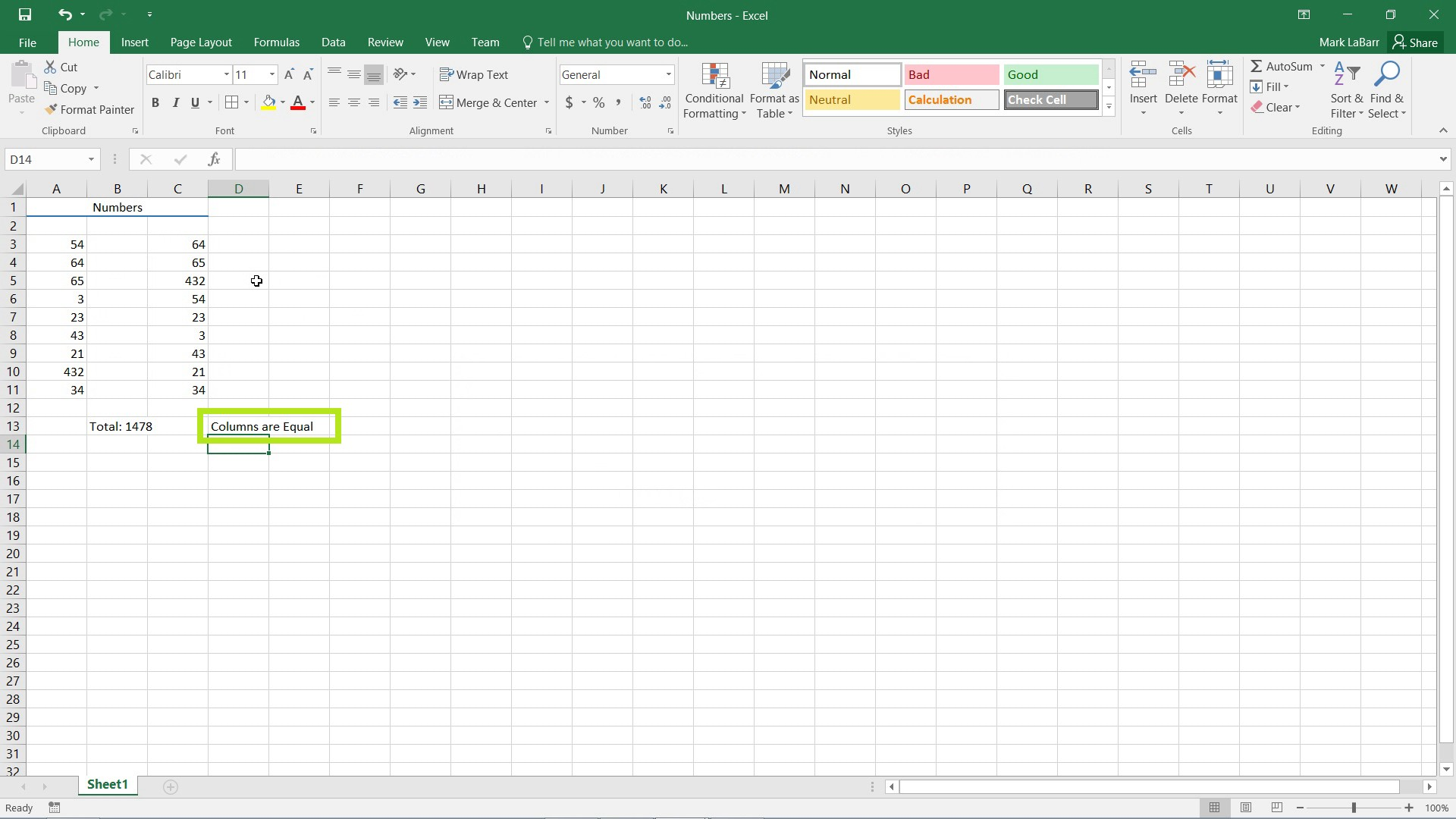## How to use the IF Function in Excel 2016

In this tutorial, we’re going to show you how to use the IF function in Excel 2016.

First, select the cell in which you want the IF function. Type “=IF” (without parentheses) in the cell itself or in the formula bar above.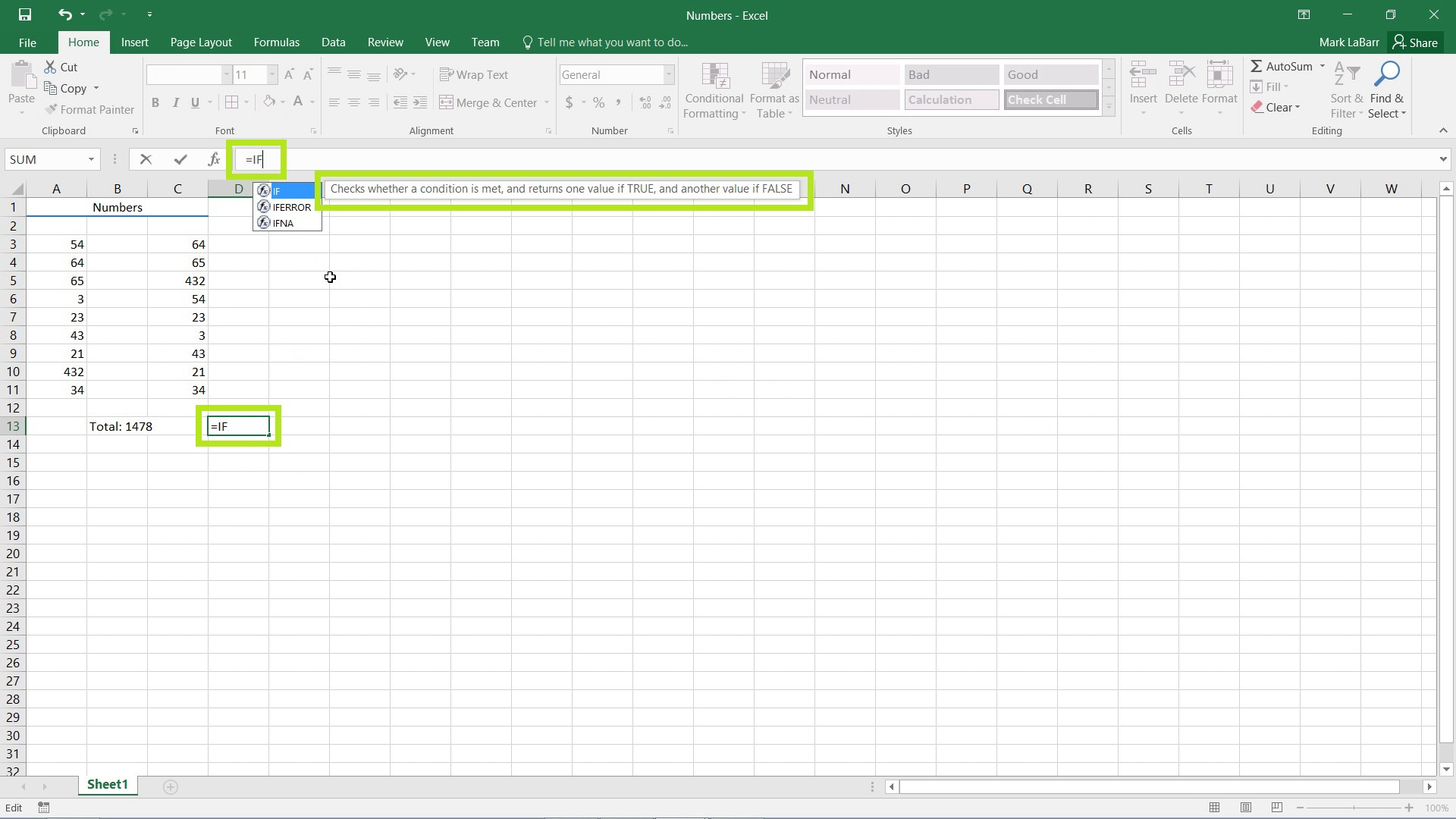Placing a “(“ parentheses sign as shown below after “=IF” brings up a tooltip. To use the IF function, you will see that we need a logical test, and what to display if the value is true or false.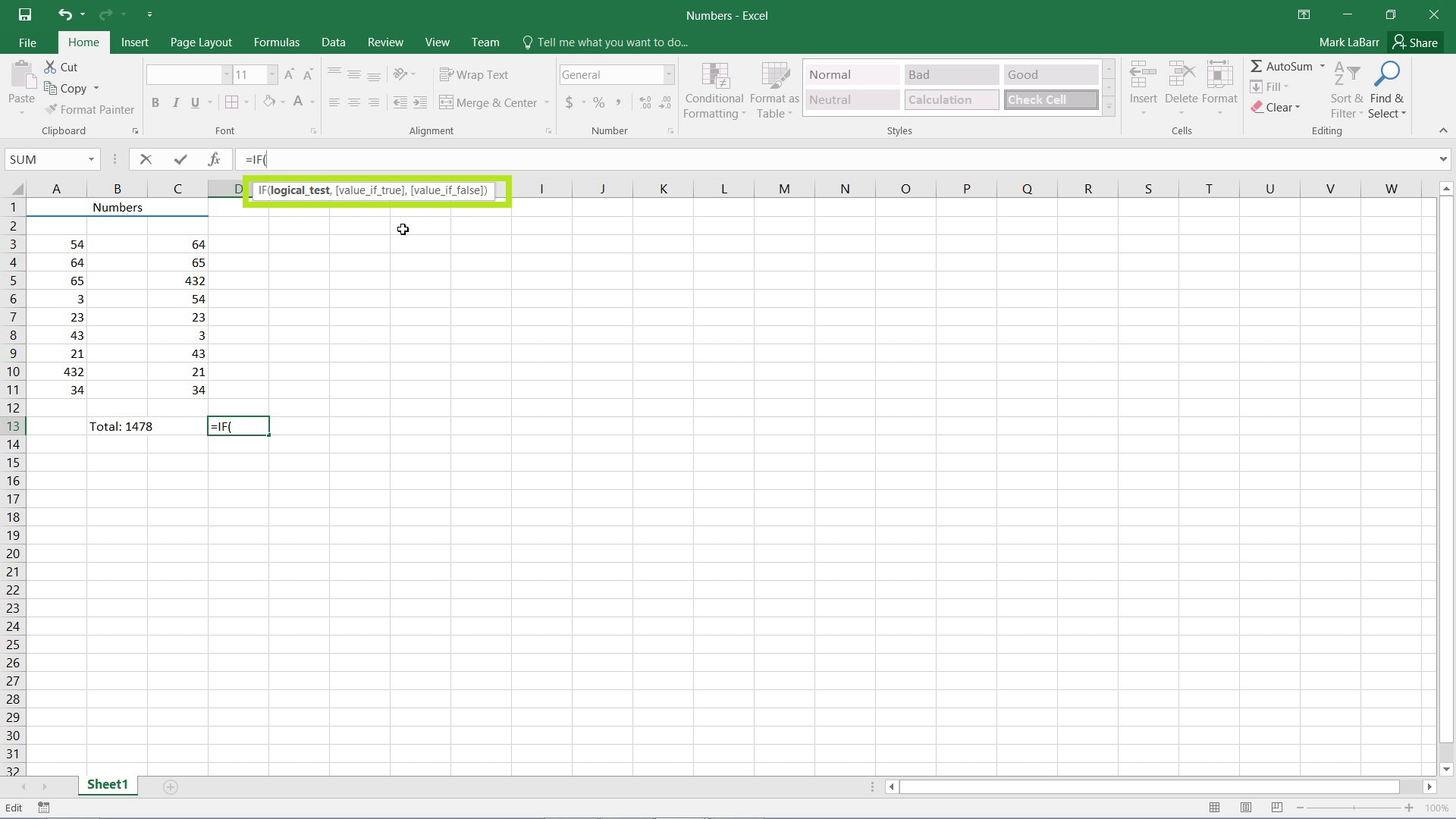In this example, we want to test and see if the sum of the left column is greater than the sum of the right column. To do this, we must nest a function inside a function. Call the SUM function inside the IF function as shown below. “=IF(SUM(A3:A11) > SUM(C3:C11)”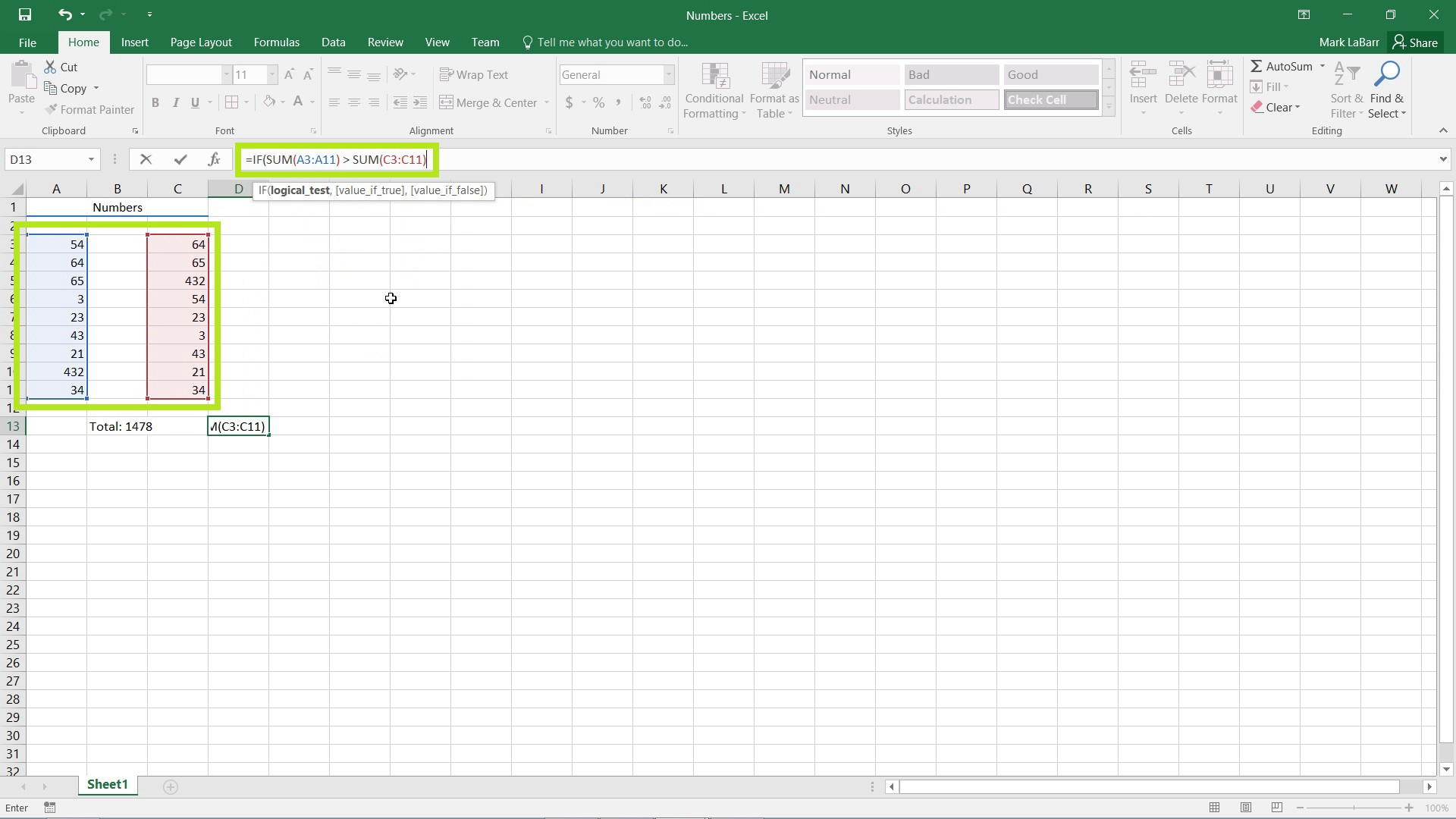Place a comma after what we’ve typed so far, and the next argument that we need is bolded in the tooltip, a value if true.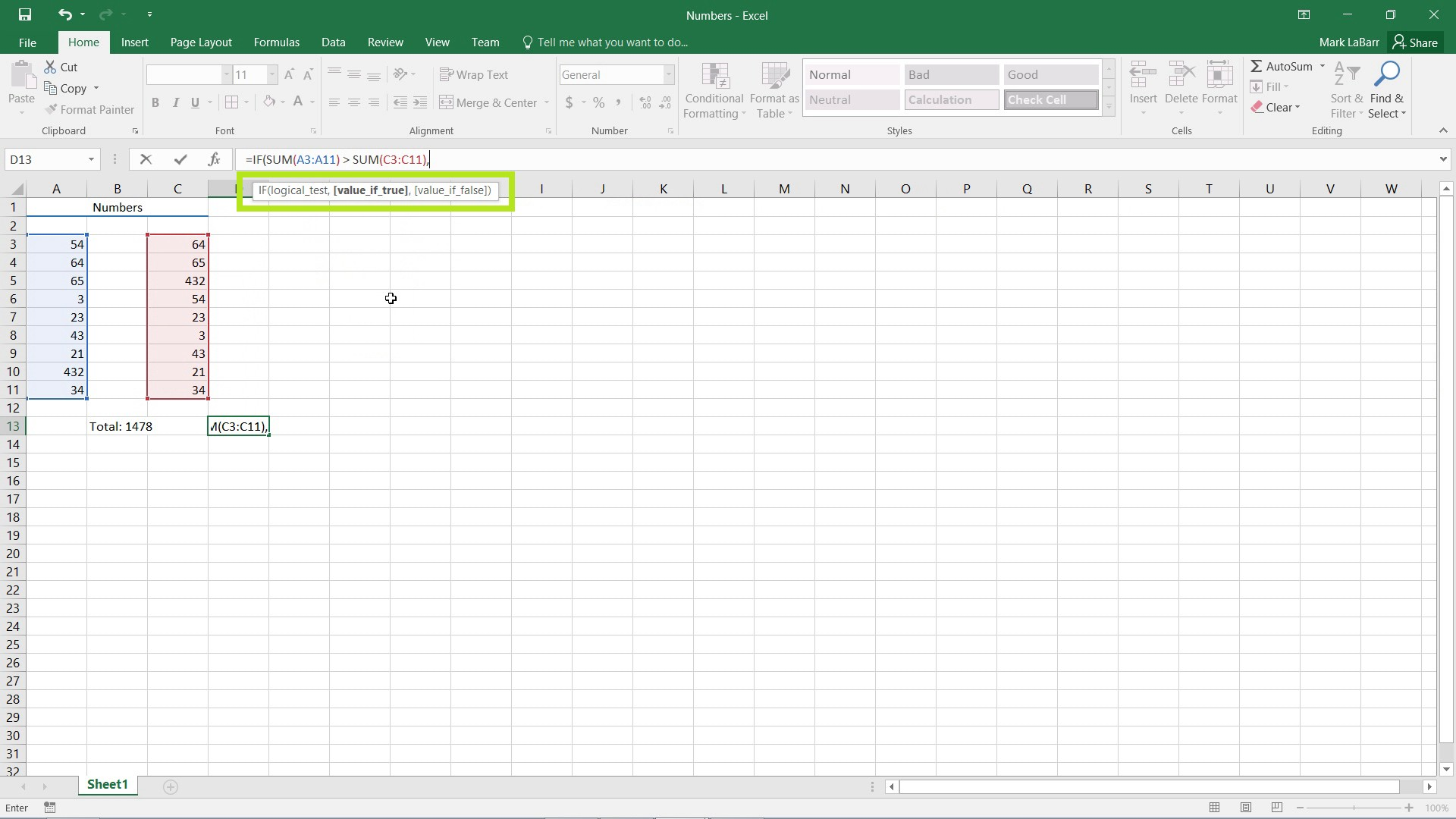If our logical test, if the sum of the left column is greater than the right, is true, we want the cell to display “Left Column Greater Than Right”. Type this in, with the parentheses because it is a string value and put a comma afterwards (outside the quotes).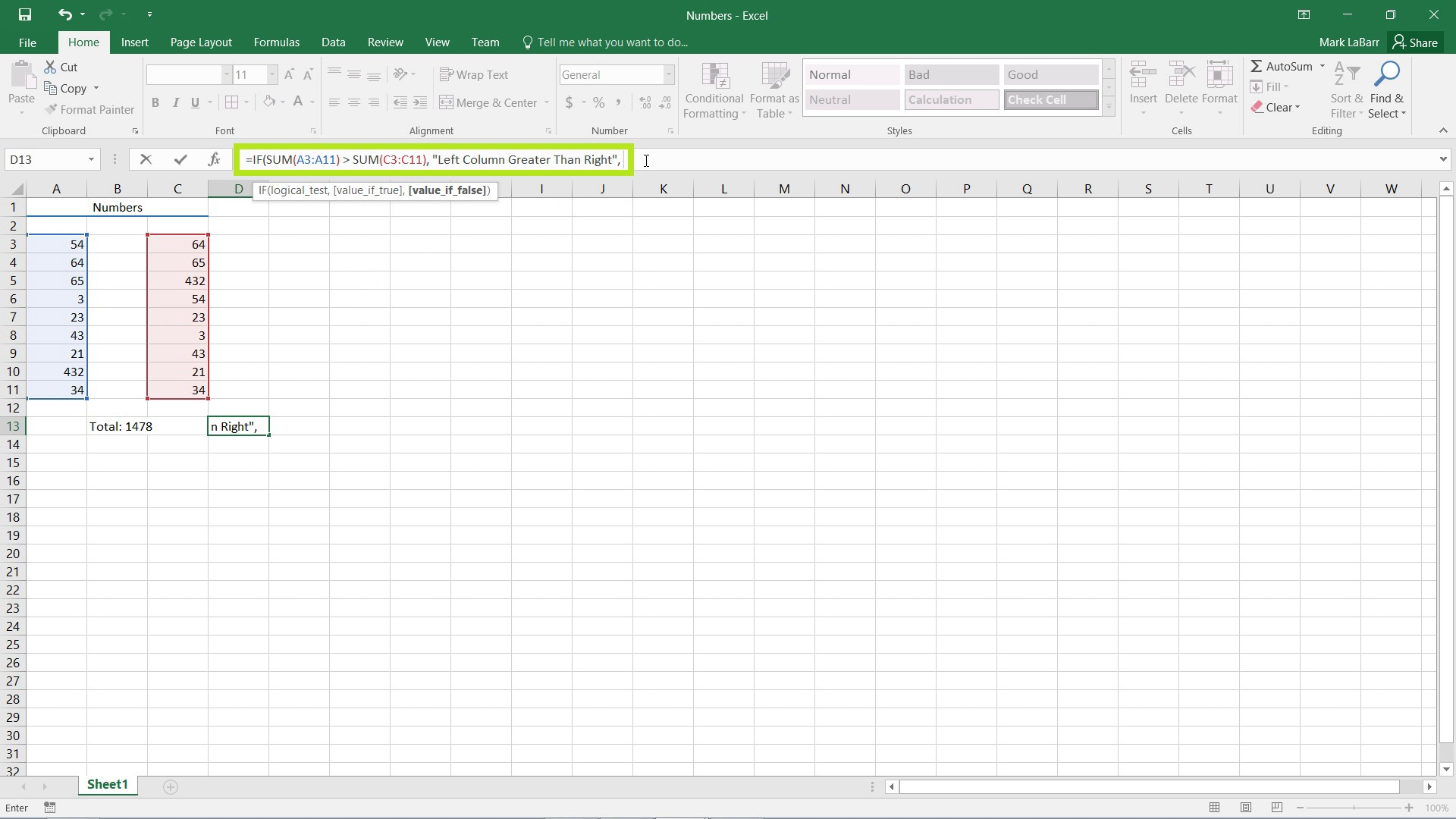The IF function is good and all, however if we wanted extra functionality, say an option for the columns to be equal, we need more than two options: true or false. To solve this, we need to nest an IF function inside our IF function. If the left column is NOT greater than the right, we need to do a check if they are equal or the right is greater than the left. For the value if false argument of the first IF formula, type “IF(“ without the parentheses to start another IF statement.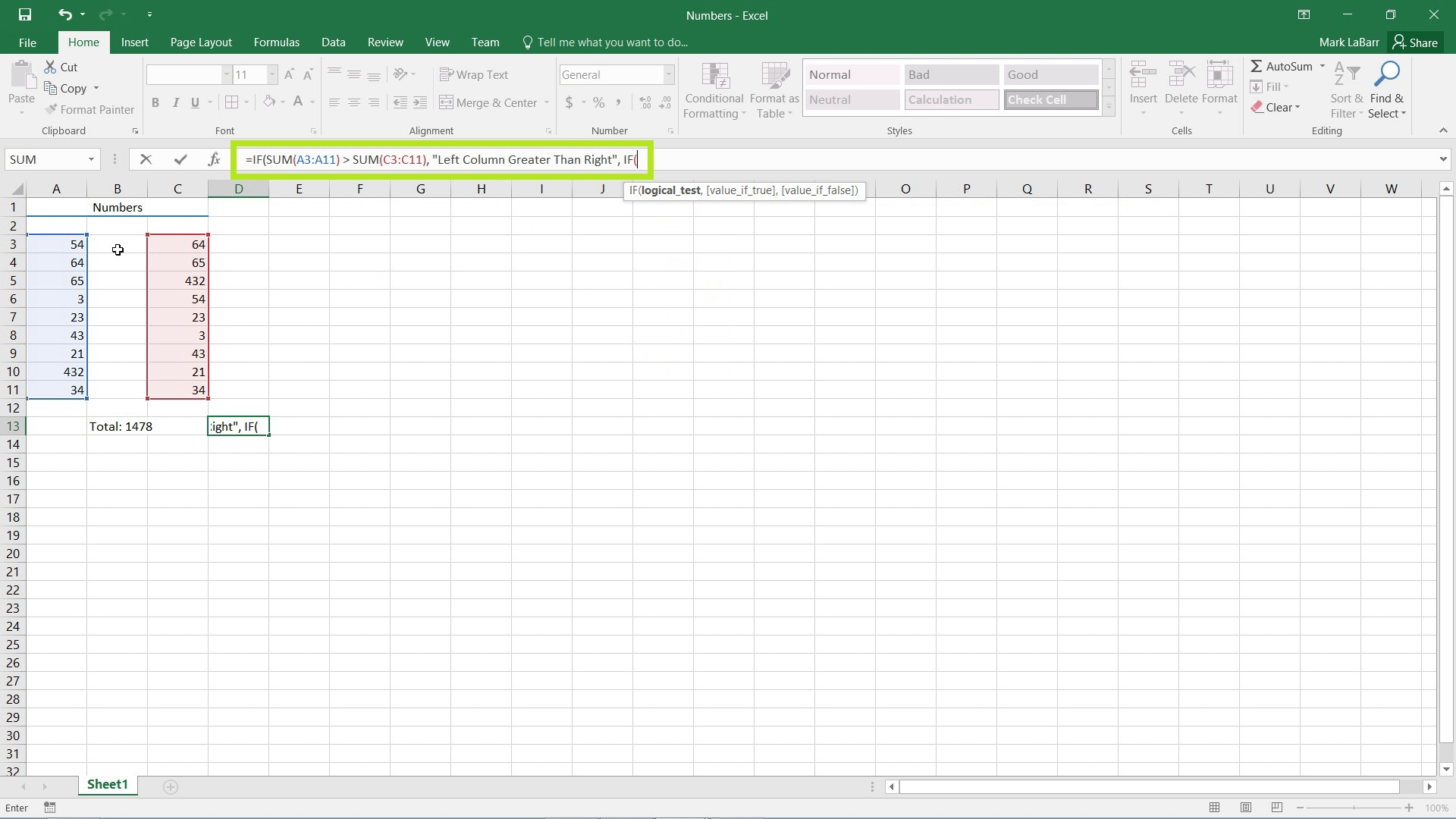For the logical test of our second IF statement, it is the same as the first except I replaced the > sign with an = sign.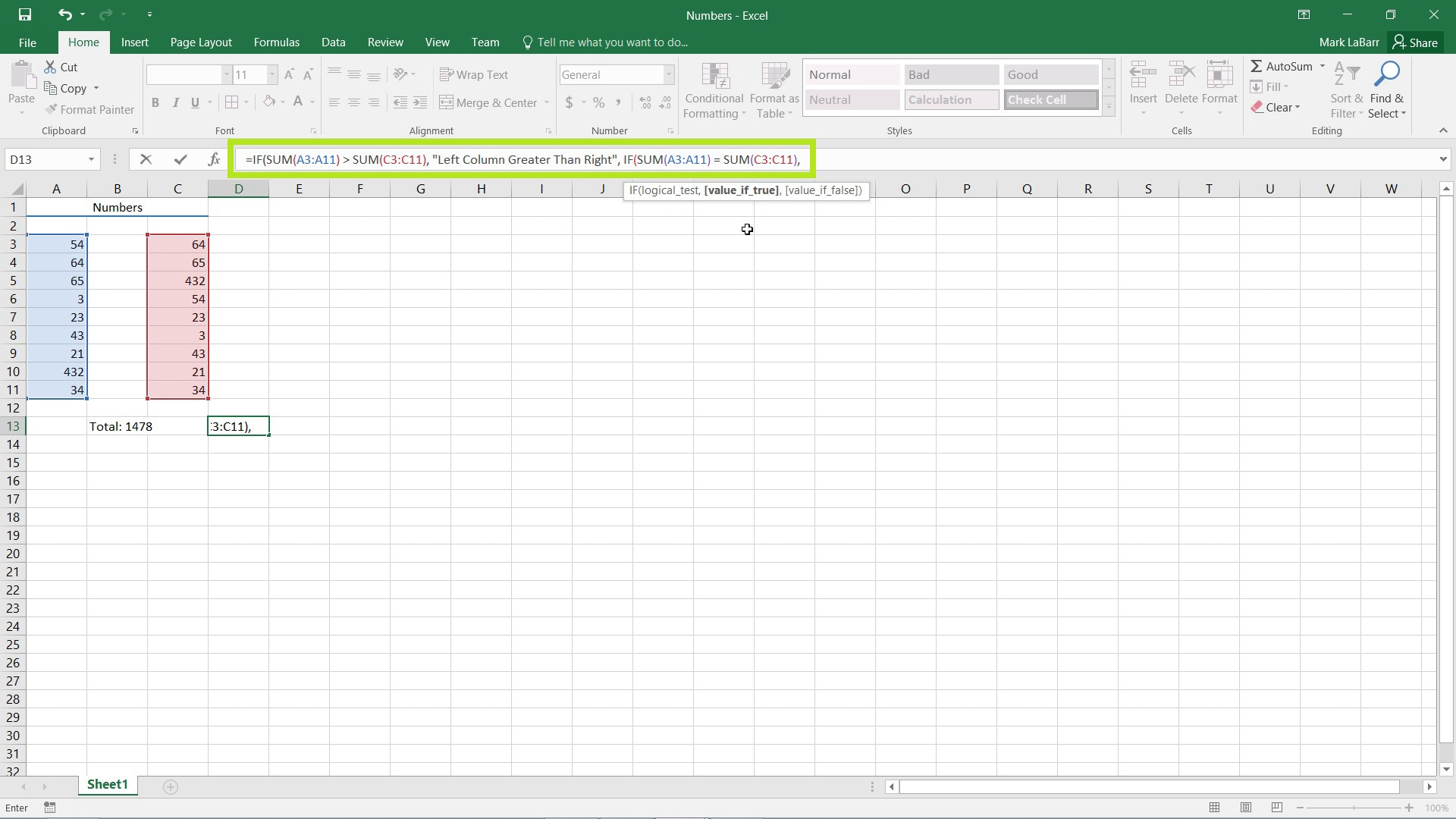So if both columns are equal, I want the cell to display “Columns are Equal” as typed in below in the example.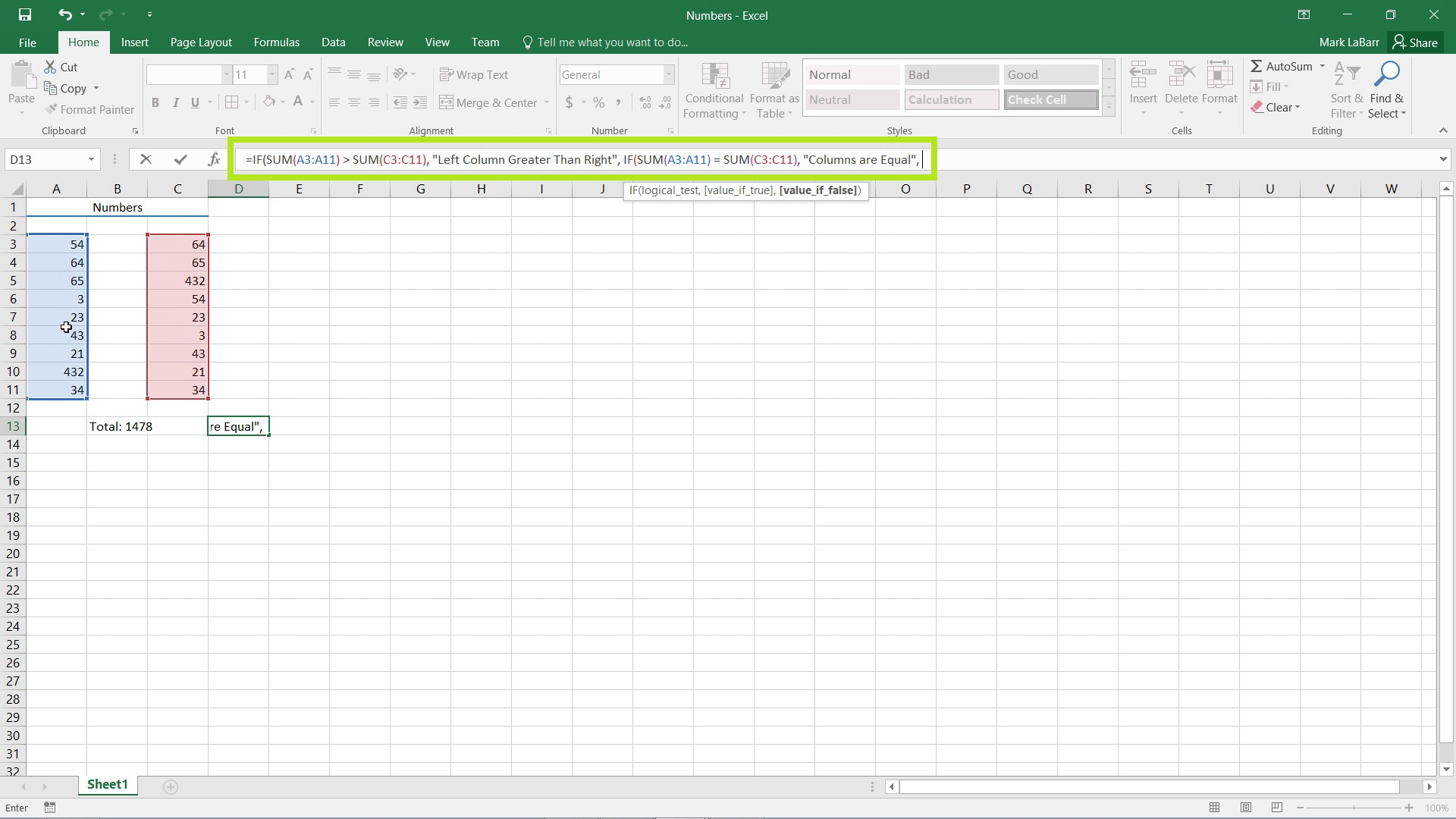However, if they are not equal, there is only one option left: that the Right Column is Greater than the Left. Close the second IF statement with a closing parentheses. And notice I left the first IF statement unclosed, Excel should pick this error up.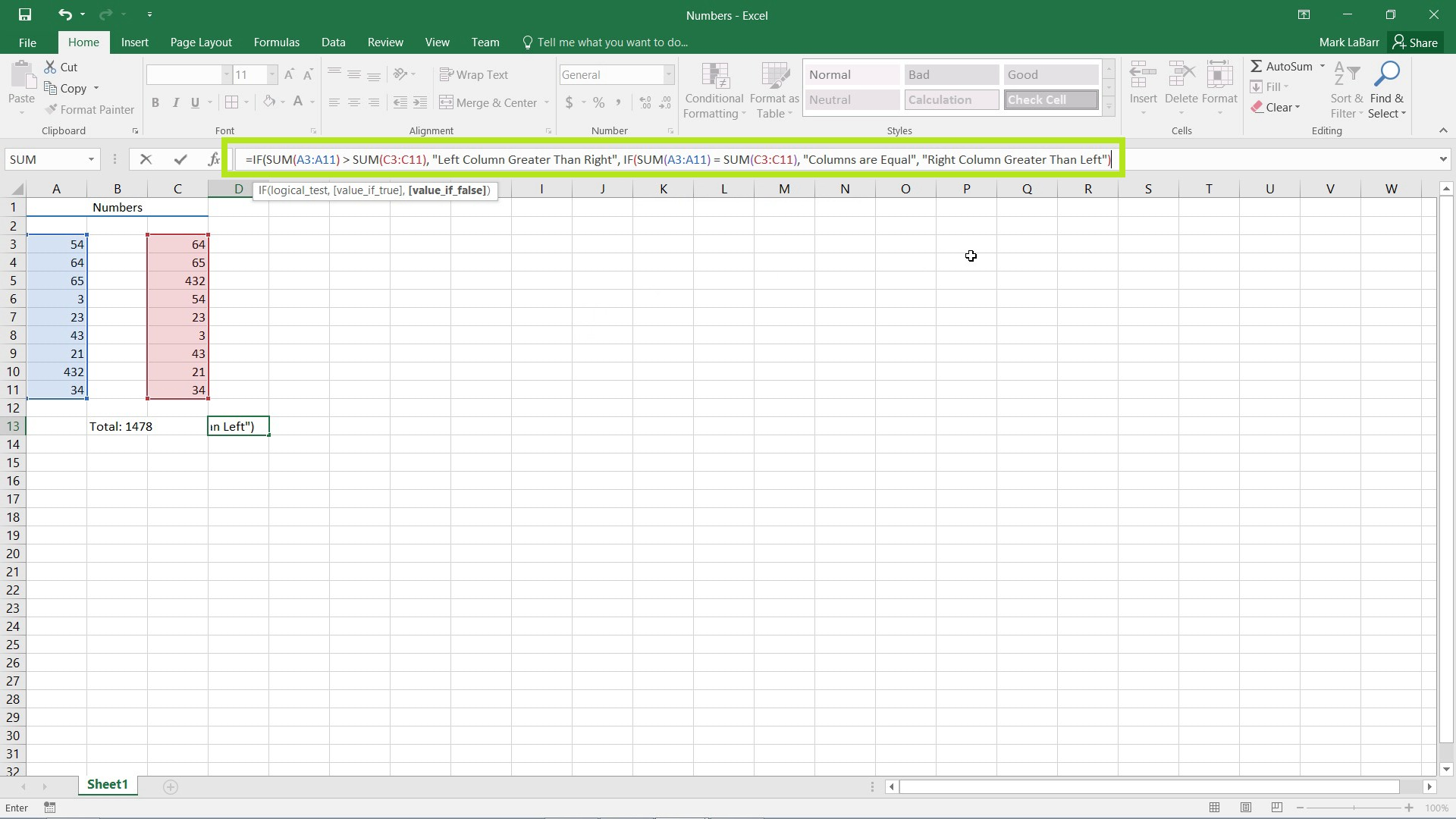Hit Enter on the keyboard and it will notify you that there is an error and ask if you wish to change it. Excel should pick up simple mistakes like this for you.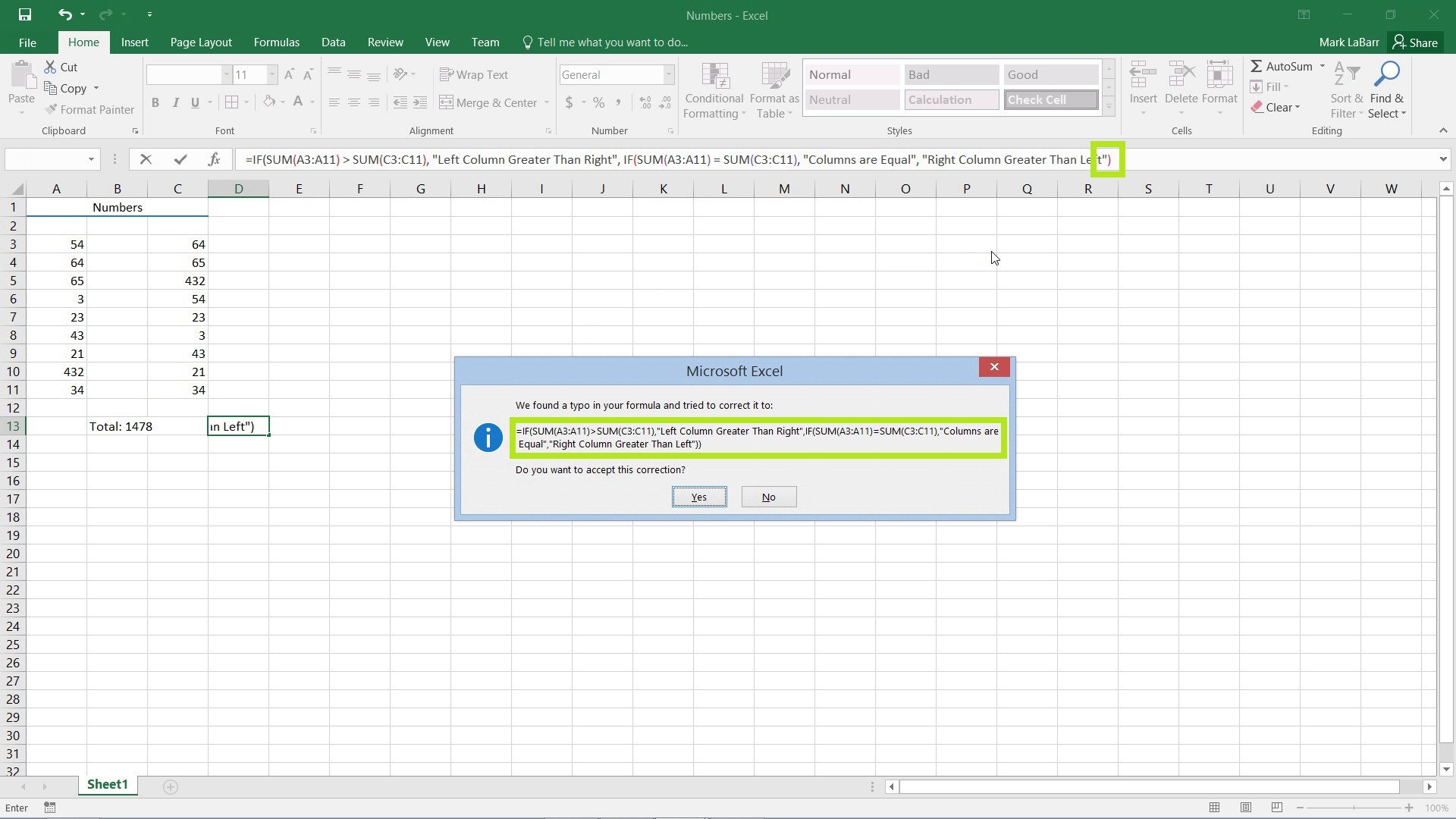When the formula is correct, the cell should output the proper text. In the example below, both columns are equal, and the cell is displaying the correct text.